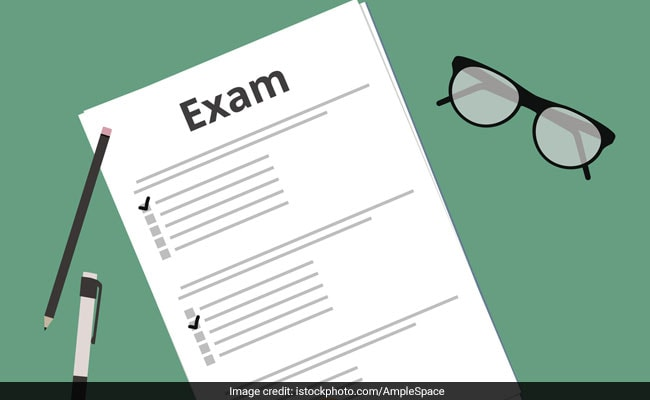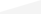CBSE Class 12 Chemistry Paper: Last Minute Preparation Tips

CBSE class 12 Chemistry paper will be held tomorrow.

Share
EMAIL
PRINTCBSE Class 12 Chemistry Paper Expert Tips

New Delhi:

Class 12 students of all CBSE affiliated schools will appear for the Chemistry paper tomorrow. One of the main subjects, Chemistry, is a compulsory paper for the science students. The exam will carry a total of 70 marks and will be of three hours duration. The highest marks per question will be 5 and all the three questions carrying 5 marks each will have internal choice options. There will also be questions carrying 2 marks and 3 marks. There will be 5 questions carrying 1 mark each and candidates have to answer them briefly.

CBSE Class 12 Students Start Online Petition; Demand Lenient Checking For Accountancy Paper

The questions involving mathematical deductions should be answered carefully. Each step carries 0.5-1 mark. For example, to answer a question on calculating the freezing point of a solution, the student should assign the variables write the formula next and then the calculation. The formula carries 0.5 marks in a 2 mark question.

CBSE Students To Soon Have Lessons Through Music, Dance And Arts

For 5 mark questions, neatly drawn and labelled figures and tables should be added wherever necessary.

Students should also take care of the presentation of answers. Proper space should be given between two consecutive answers; a properly drawn line can also help the examiner distinguish between two answers.

CBSE Concludes Class 12 Sociology Paper; Students Call It Moderate

Each of the answers should be marked with the respective question number circled or written in bold.

In case a question has been left, the student should attempt it later by mentioning the question number and the serial number of the question, if required.

Do revise the answer paper at least once, before handing it over to the invigilator.